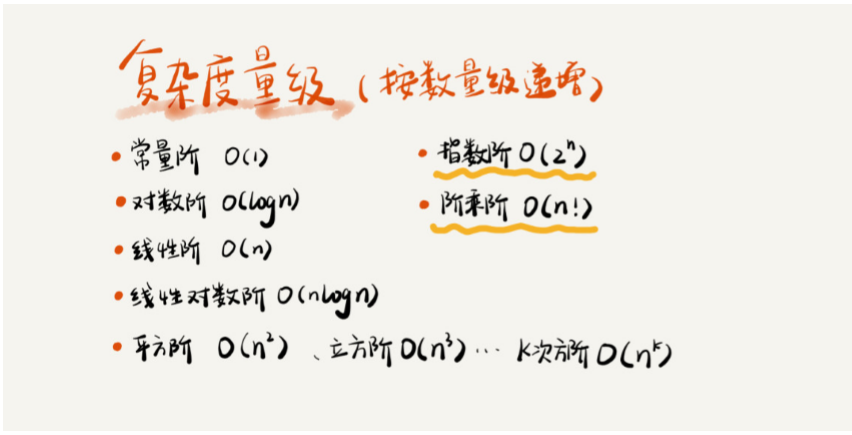# 如何分析，统计算法的执行效率和资源消耗？

1.测试结果非常依赖测试环境

2.测试结果受着数据规模的影响很大

int cal(int n) {
int sum = 0;
int i = 1;
for (; i <= n; ++i) {
sum = sum + i;
}
return sum;
}


int cal(int n) {
int sum =0;
int i = 1;
int j = 1;
for (; i <= n; ++i) {
j = 1;
for(; j <= n; ++j) {
sum = sum + i * j;
}
}
}


1.只关注循环执行次数最多的一段代码

int cal(int n) {
int sum = 0;
int i = 1;
for (; i <= n; ++i) {
sum = sum + i;
}
return sum;
}


2.加法法则：总复杂度等于量级最大的那段代码的复杂度

int cal(int n)
{
int sum_1 = 0;
int p = 1;
for (; p < 100; ++p) {
sum_1 = sum_1 + p;
}
int sum_2 = 0;
int q = 1;
for (; q < n; ++q) {
sum_2 = sum_2 + q;
}
int sum_3 = 0;
int i = 1;
int j = 1;
for (; i <= n; ++i) {
j = 1;
for (; j <= n; ++j) {
sum_3 = sum_3 +  i * j;
}
}
return sum_1 + sum_2 + sum_3;
}


3.乘法法则：嵌套代码的复杂度等于嵌套内外代码复杂度的乘积

	int cal(int n) {
int ret = 0;
int i = 1;
for (; i < n; ++i) {
ret = ret + f(i);
}
}

int f(int n) {
int sum = 0;
int i = 1;
for (; i < n; ++i) {
sum = sum + i;
}
return sum;
}1.O(1)

 int i = 8;
int j = 6;
int sum = i + j;


2.O(logn)、O(nlogn)

 i=1;
while (i <= n) {
i = i * 2;
}


	**2^0^		2^1^    2^2^ ...2^k^ ... 2^x^ = n**


i=1;
while (i <= n)  {
i = i * 3;
}


3. O(m+n)、O(m*n)

int cal(int m, int n) {
int sum_1 = 0;
int i = 1;
for (; i < m; ++i) {
sum_1 = sum_1 + i;
}
int sum_2 = 0;
int j = 1;
for (; j < n; ++j) {
sum_2 = sum_2 + j;
}
return sum_1 + sum_2; }


02-1761710-29139
06-22351
04-10426
07-044246
09-26156
10-101168
08-235032
10-281532
09-243067
03-0919
02-142059
08-05490
10-18257
04-19315
07-022100
03-311228
11-072279
11-1332
©️2020 CSDN 皮肤主题: 深蓝海洋 设计师:CSDN官方博客